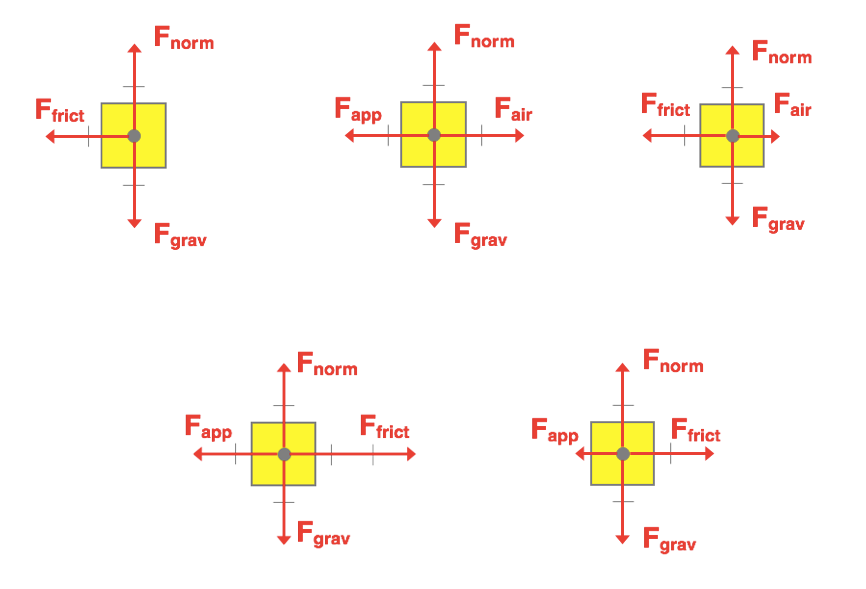Change of State

The Change of State Concept Builder is comprised of 12 situations in which a free body diagram is provided for a given state of motion and learners must decide how the individual forces will change in order for the object's state of motion to change. Learners select the new free body diagram (Force Diagram) from a set of five choices. There are two levels of difficulty by which the student can progress through the Concept Builder. Those difficulty levels are differentiated as follows:

• Masters Level: Situations 1-6
• Wizard Level: Situations 1-12

The additional six situations  of the Wizard Level may be slightly more complicated than the six situations of the Masters Level. The situations are shown below - complete with the given graph and the graph options for the new state of motion. Teachers are encouraged to view the questions in order to judge which level of difficulty is most appropriate for their classes.

The Physics Classroom grants teachers and other users the right to print these questions for private use. Users are also granted the right to copy the text and modify it for their own use. However, this document should not be uploaded to other servers for distribution to and/or display by others. The Physics Classroom website should remain the only website or server from which the document is distributed or displayed. We also provide a PDF that teachers can use under the same conditions. We have included a link to the PDF near the bottom of this page.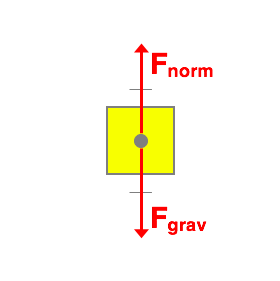Question 1:
An elevator rider is moving upward at a constant speed. The Force Diagram for this state of motion is shown on the left. What will the Force Diagram look like when this upward-moving rider undergoes a slowing down process? Select a force diagram from the choices provided on the right.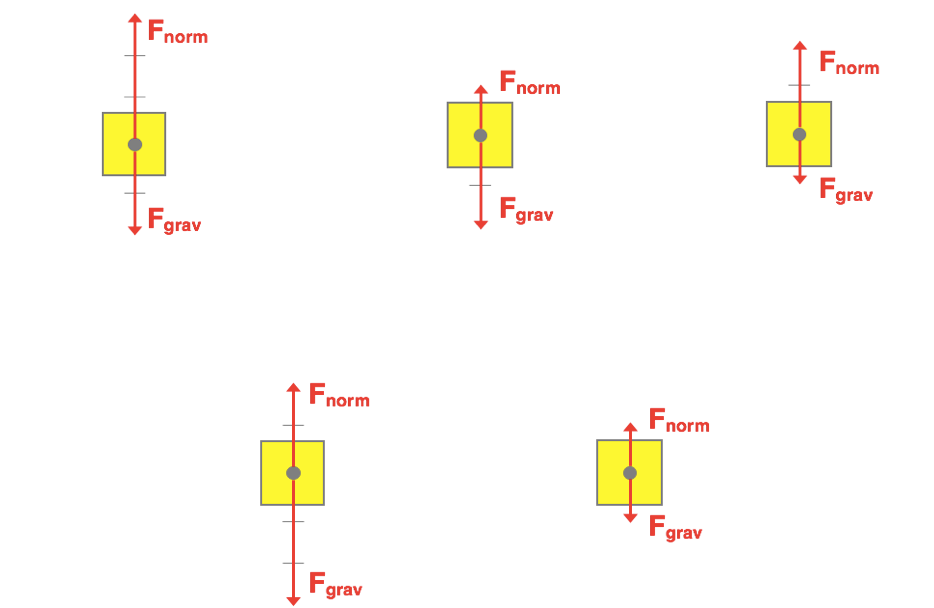Question 2:
An elevator rider is at rest on the ground floor. The Force Diagram for this state of motion is shown on the left. What will the Force Diagram look like when this rider is in a state of moving upward with an increasing speed? Select a force diagram from the choices provided on the right.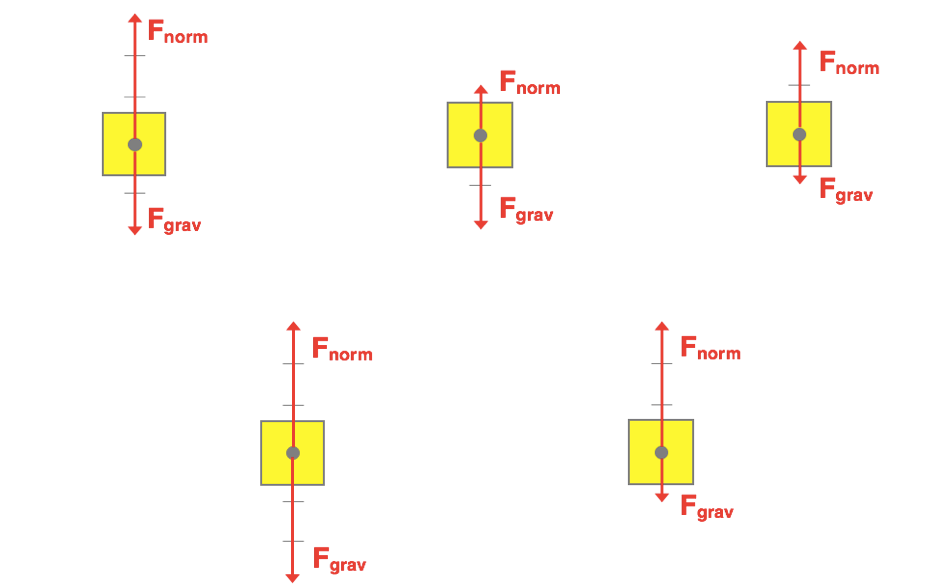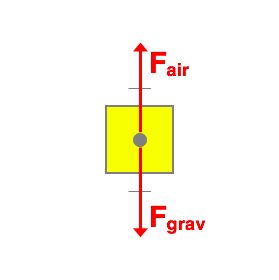Question 3:
A disk is moving rightward at a constant speed across a 'no-friction' air table. The Force Diagram for this state of motion is shown on the left. What will the Force Diagram look like if the air is turned off and this rightward-moving disk is in the state of slowing down? Select a force diagram from the choices provided on the right.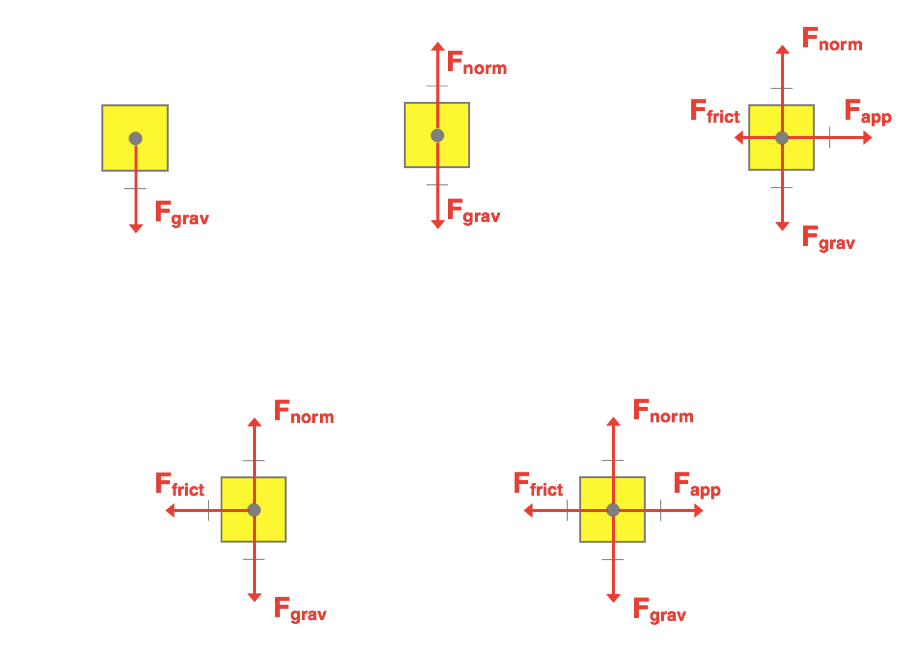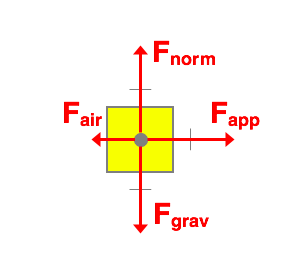Question 4:
A rightward-moving car is speeding up. The Force Diagram for this state of motion is shown on the left. What will the Force Diagram look like if the driver eases off slightly on the gas pedal and maintains a constant speed? Select a force diagram from the choices provided on the right.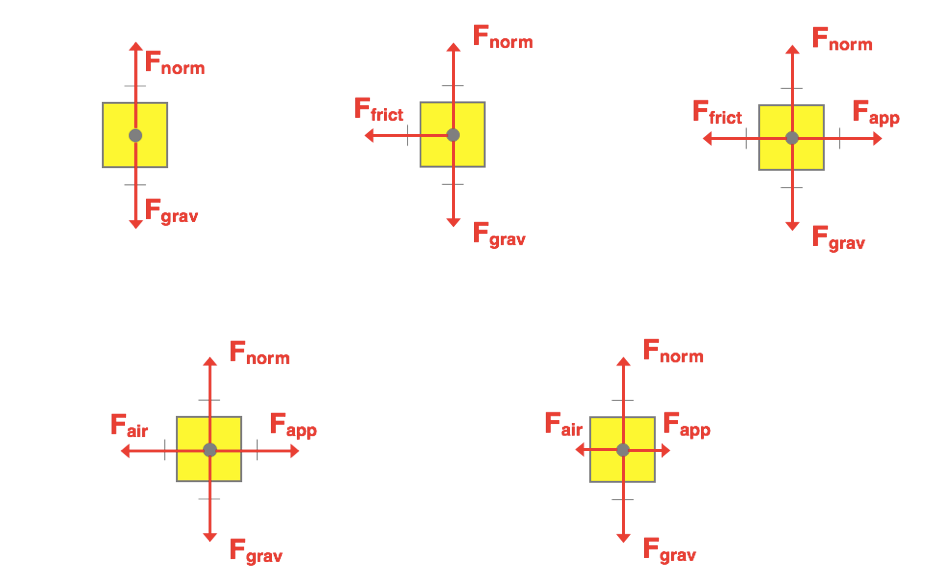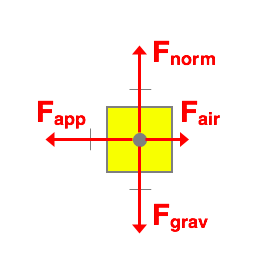Question 5:
A leftward-moving car is speeding up. The Force Diagram for this state of motion is shown on the left. What will the Force Diagram look like if the driver eases off slightly  on the gas pedal and maintains a constant speed? Select a force diagram from the choices provided on the right.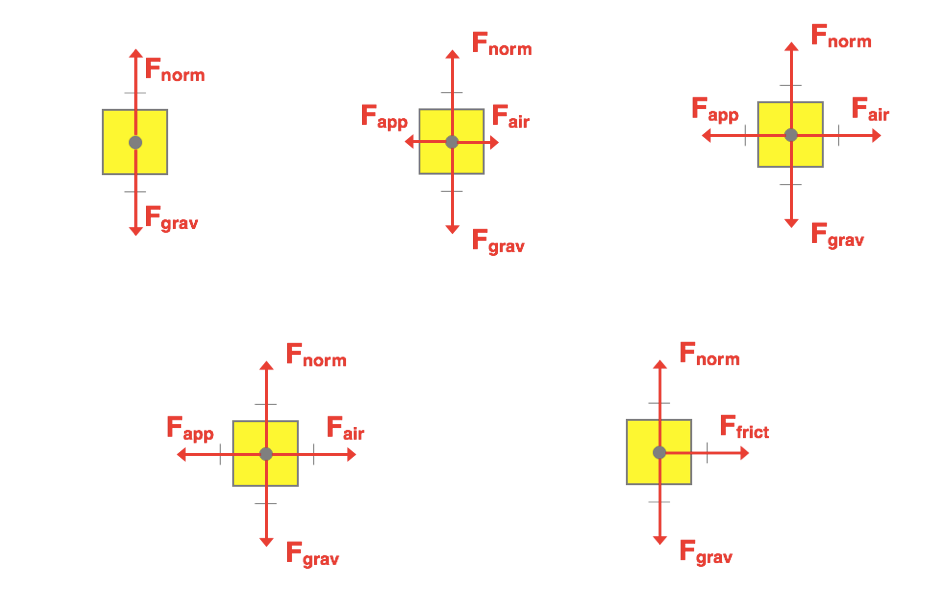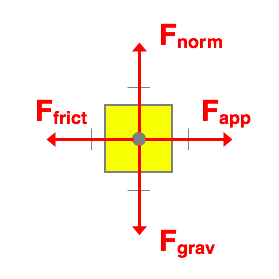Question 6:
A worker is applying a force on a large crate to move it rightward at a constant speed. The Force Diagram for this state of motion is shown on the left. What will the Force Diagram look like if the worker stops pushing and the rightward-moving crate is in a state of slowing down? Select a force diagram from the choices provided on the right.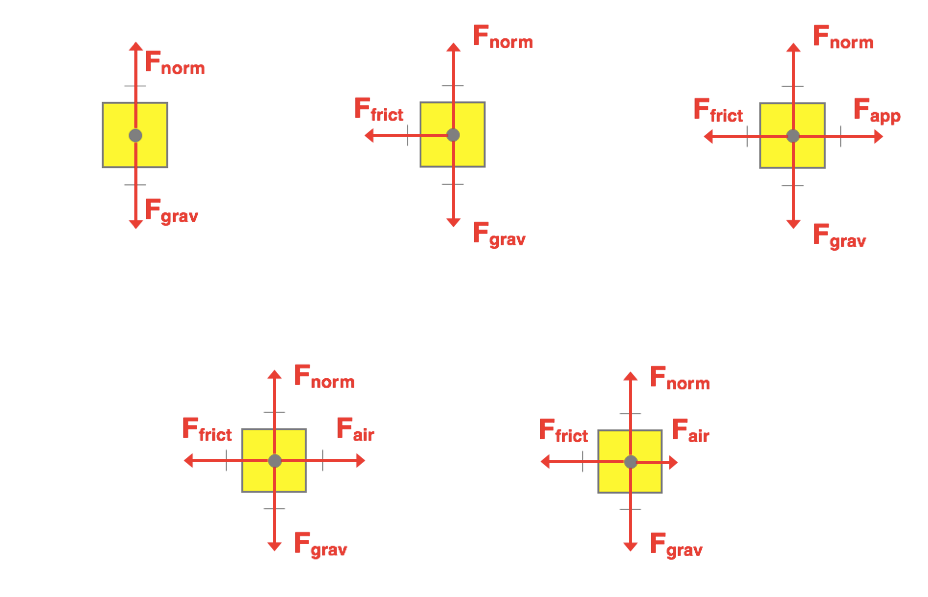Question 7:
An elevator rider is moving downward at a constant speed. The Force Diagram is shown. What will the Force Diagram look like when this downward-moving rider undergoes a slowing down process? Select a force diagram from the choices provided on the right.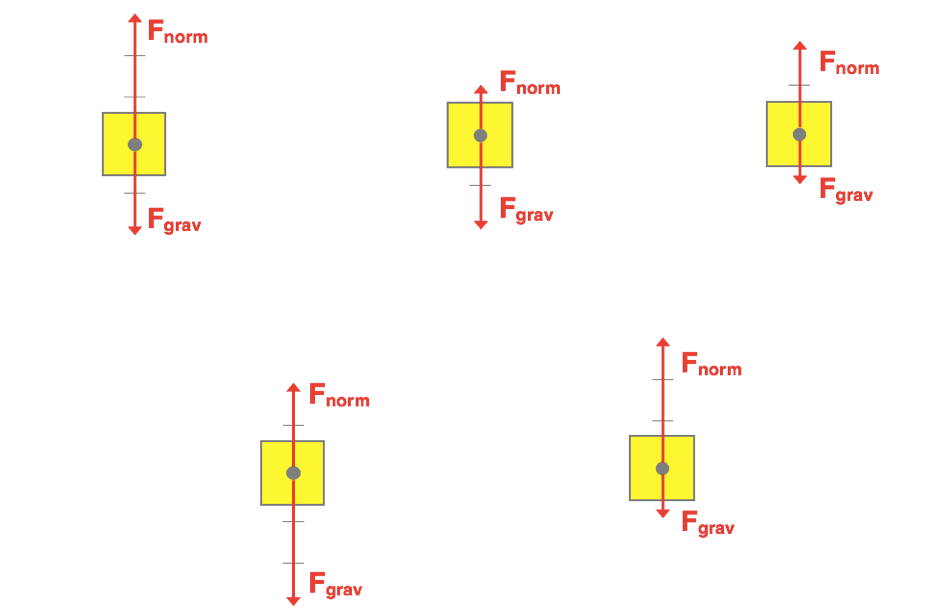Question 8:
An elevator rider is at rest on the top floor. The Force Diagram is shown. What will the Force Diagram look like when this rider is in a state of moving downward with an increasing speed? Select a force diagram from the choices provided on the right.Question 9:
A disk at moving leftward at a constant speed across a 'no-friction' air table. The Force Diagram is shown. What will the Force Diagram look like if the air is turned off and this leftward-moving disk is in the state of slowing down? Select a force diagram from the choices provided on the right.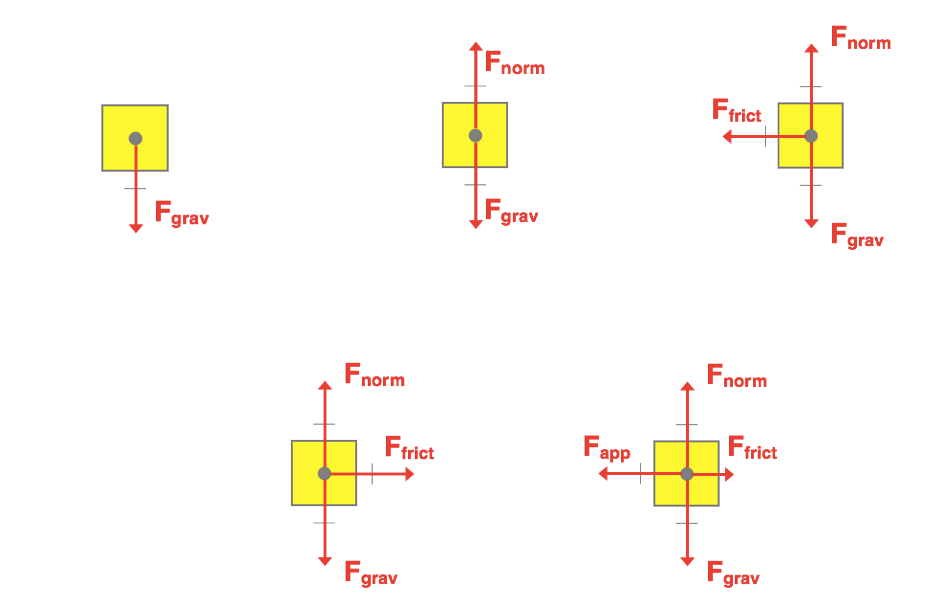Question 10:
A skydiver is falling downward at a constant speed. The Force Diagram is shown. What will the Force Diagram look like if she reorients herself and is in a state of slowing down? Select a force diagram from the choices provided on the right.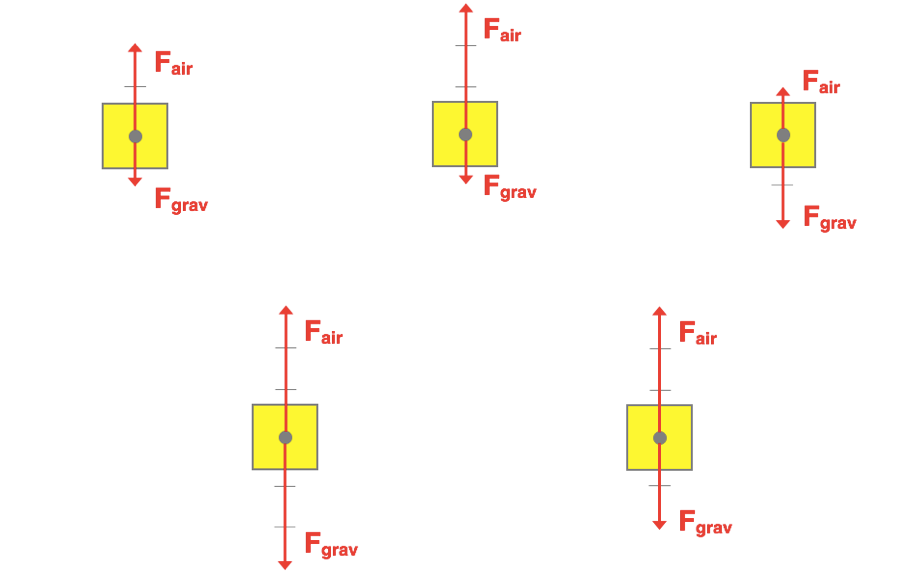Question 11:
A skydiver is falling downward at a constant speed. The Force Diagram is shown. What will the Force Diagram look like if she reorients herself and is in a state of speeding up? Select a force diagram from the choices provided on the right.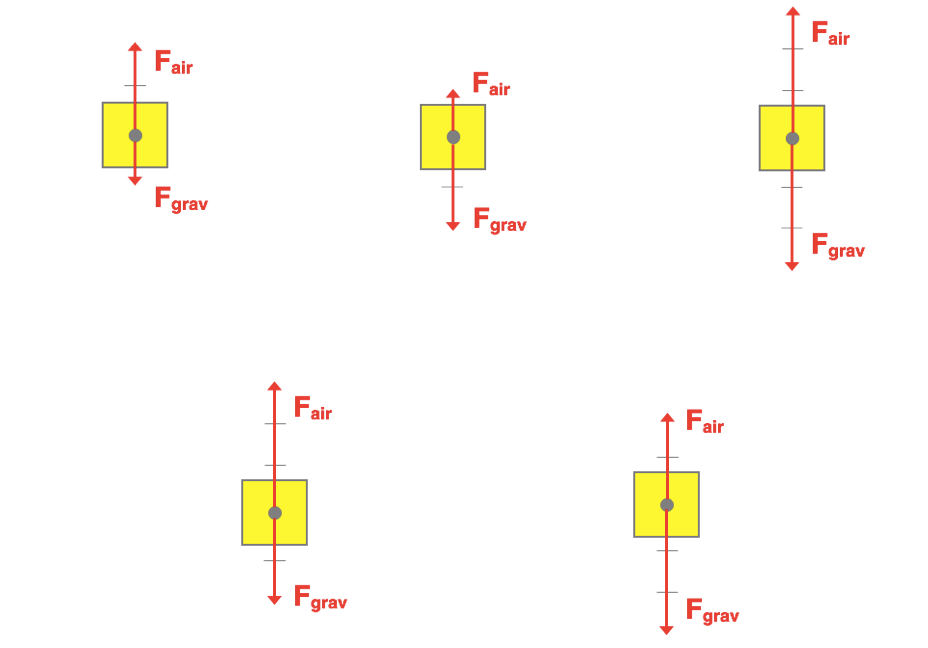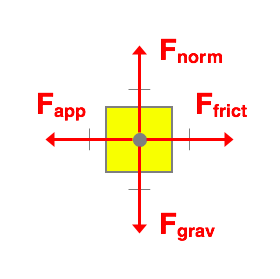Question 12:
A worker is applying a force on a large crate to move it leftward at a constant speed. The Force Diagram is shown. What will the Force Diagram look like if the worker stops pushing and the leftward-moving crate is in a state of slowing down? Select a force diagram from the choices provided on the right.